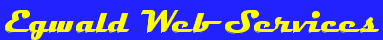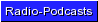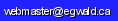Egwald Mathematics — Nonlinear Dynamics — Systems of Equationsby Elmer G. Wiens Egwald's popular web pages are provided without cost to users. Please show your support by joining Egwald Web Services as a Facebook Fan:Follow Elmer Wiens on Twitter:Two Dimensional Flows and Phase Diagrams Introduction to two dimensional flows. 1. Dynamics of linear systems of differential equations. 2. Classification of Fixed Points for Linear Systems: stability diagram. 3. The general form of a two dimensional nonlinear system of equations. 4. Determining the fixed points of a two dimensional nonlinear system. 5. The linearized stability of a two dimensional nonlinear system. 6. The Jacobian of a two dimensional nonlinear system. 7. Classification of Fixed Points for Linear Systems: topological diagram. 8. Example of a nonlinear dynamic system: Strogatz example. 9. Example of a nonlinear dynamic system: Boyce and DiPrima. 10. Example of a nonlinear dynamic system: oscillating pendulum. 11. The dynamics diagram of the oscillating pendulum. 12. The Lotka � Volterra � Goodwin predator-prey model. Limit Cycles and Two Dimensional Flows Introduction to limit cycles. 1. Example of a nonlinear system with a limit cycle: example. 2. Transformation to polar coordinates. 3. The van der Pol Oscillator as the prototype of a nonlinear system exhibiting limit cycles. 4. Criteria to determine the absence or presence of limit cycles. 5. The Poincaré-Bendixson Theorem. 6. The Kaldor Business Cycle Model. Bifurcations and Two Dimensional Flows Introduction to bifurcations. 1. Prototype bifurcations:           a. saddle-node bifurcation.           b. transcritical bifurcation.           c. pitchfork bifurcation. 2. In Hopf bifurcations, a stable limit cycle emerges — growing from a fixed point (supercritical Hopf bifurcation), or an unstable limit cycle disappears — diminishing towards a fixed point. 3. Two parameter bifurcations and the monkey saddle bifurcation as an example. 4. Global bifurcations:           a. global saddle-node bifurcation.           b. infinite period bifurcation.           c. homoclinic bifurcation. Mathematical Appendix Introduction to mathematical appendix. 1. The direction field and phase portrait provide qualitative information about the solutions to a system of differential equations. 2. The fixed points (equilibrium points) of the nonlinear differential equation system. 3. Fixed points occur at the intersection(s) of the system's nullclines. 4. Nonlinear dynamical systems can have multiple fixed points which may be stable or unstable. 5. The Taylor series expansion of the equations of motion linearizes the system about its fixed points. 6. The Jacobian matrix reveals the dynamics of the nonlinear system. 7. Saddle points may have homoclinic or heteroclinic orbits (solution trajectories). 8. A differential equation system with a first integral is called a conservative system. 9. The Lotka—Volterra predator-prey model as an example of a conservative system. 10. Use index theory to detect the presence and characteristics of fixed points.Copyright © Elmer G. Wiens:   Egwald Web ServicesAll Rights Reserved.    Inquiries